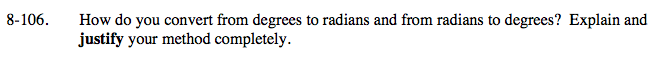### Home > A2C > Chapter 8 > Lesson 8.1.7 > Problem8-106

8-106.Notice that when you know the number of radians in π form, you can convert to degrees byletting π = 180°.
Show some examples.

$\text{To convert from radians to degrees, multiply by }\frac{180}{\pi}.$

Show some examples.

$\text{In all situations you can simply set up a proportion with } \frac{\pi \text{ radians}}{180\degree} \text{ as one of the ratios.}$

Show some examples.